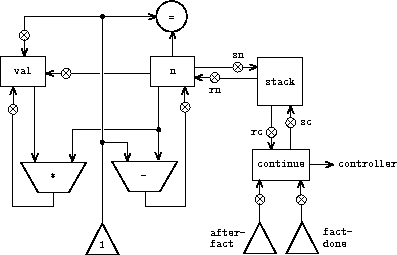# The quality of the compiler

By comparing the stack operations used by compiled code to the stack operations used by the evaluator for the same computation, we can determine the extent to which the compiler optimizes use of the stack, both in speed (reducing the total number of stack operations) and in space (reducing the maximum stack depth). Comparing this optimized stack use to the performance of a special-purpose machine for the same computation gives some indication of the quality of the compiler.

a. Exercise 5.27 asked you to determine, as a function of n `n` , the number of pushes and the maximum stack depth needed by the evaluator to compute n! `n!` using the recursive factorial procedure given above. Exercise 5.14 asked you to do the same measurements for the special-purpose factorial machine shown in figure 5.11. Now perform the same analysis using the compiled factorial `factorial` procedure.

Take the ratio of the number of pushes in the compiled version to the number of pushes in the interpreted version, and do the same for the maximum stack depth. Since the number of operations and the stack depth used to compute n! `n!` are linear in n `n` , these ratios should approach constants as n `n` becomes large. What are these constants? Similarly, find the ratios of the stack usage in the special-purpose machine to the usage in the interpreted version.

Compare the ratios for special-purpose versus interpreted code to the ratios for compiled versus interpreted code. You should find that the special-purpose machine does much better than the compiled code, since the hand-tailored controller code should be much better than what is produced by our rudimentary general-purpose compiler.

b. Can you suggest improvements to the compiler that would help it generate code that would come closer in performance to the hand-tailored version?``````(controller
(assign continue (label fact-done))     ; set up final return address
fact-loop
(test (op =) (reg n) (const 1))
(branch (label base-case))
;; Set up for the recursive call by saving n and continue.
;; Set up continue so that the computation will continue
;; at after-fact when the subroutine returns.
(save continue)
(save n)
(assign n (op -) (reg n) (const 1))
(assign continue (label after-fact))
(goto (label fact-loop))
after-fact
(restore n)
(restore continue)
(assign val (op *) (reg n) (reg val))   ; val now contains n(n - 1)!
base-case
(assign val (const 1))                  ; base case: 1! = 1